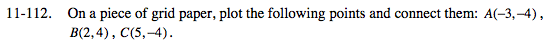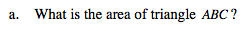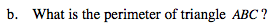Home > MC2 > Chapter 11 > Lesson 11.3.3 > Problem11-112

11-112.
1. On a piece of grid paper, plot the following points and connect them: A(–3, –4), B(2, 4), C(5, –4). Homework Help ✎

1. What is the area of triangle ABC?

2. What is the perimeter of triangle ABC?Using the etool below plot points A, B, and C onto the grid where they
belong to form the triangle.Draw a segment creating two right triangles.
Then use the Pythagorean Theorem to find
the lengths of AB and BC.

Explore using the eTool below.

Click the link at right for the full eTool version: MC2 11-112 HW eTool